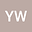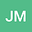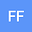Scaling Analysis of Two-Phase Flow in Fractal Permeability Fields
•••• +1Jesse Mckinzie
University of Wyoming
Author Profile## Abstract

Fluid mixing in permeable media is essential in many practical applications. The mixing process is a consequence of velocity fluctuations owing to geological heterogeneities and mobility contrast of fluids. Heterogeneities in natural rocks are often spatially correlated, and their properties, such as permeability, may be described using fractal distributions. This work models the fractal characteristics of such permeability fields in which the covariance function is expressed as a power-law function. A generalized scaling relation is derived relating various fractal permeability fields using the magnitude of their fluctuations. This relation reveals the self-similar behavior of two-phase flow in such permeable media. To that end, a recently developed, high-resolution numerical simulator is employed to validate the analytically derived scaling relations. Two flow problems are considered in which flow is governed by 1) a linear, and 2) a nonlinear transport equation. Due to the probabilistic representation of the fractal permeability fields, a sensitivity study is conducted for each flow scenario to determine the number of realizations required for statistical convergence. Scaling analysis is performed using ensemble averages of simulated saturation profiles and their mixing lengths. Results support the validity of the developed scaling relation across the range of investigated flow conditions at intermediate times. The dynamics of linear flow in the asymptotic regime is affected by the correlation structure of heterogeneity. In nonlinear flow, scaling behavior appears to be dominated by the degree of nonlinearity.
Nov 2020Published in Water Resources Research volume 56 issue 11. 10.1029/2020WR028214